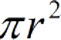Request a Tool

# Area of a Circle Calculator

Circle Area calculator use to calculate surface area of a circle.

Area
0

#### Formula• π = a constant value 3.1415
• r = radius of the Circle

#### Defination / Uses

A circle is a shape in which the distance between the centre and the circular's edge is constant. Every circle has a point in the middle.

### How calculte the area of a circle?

Its a single step procedure.